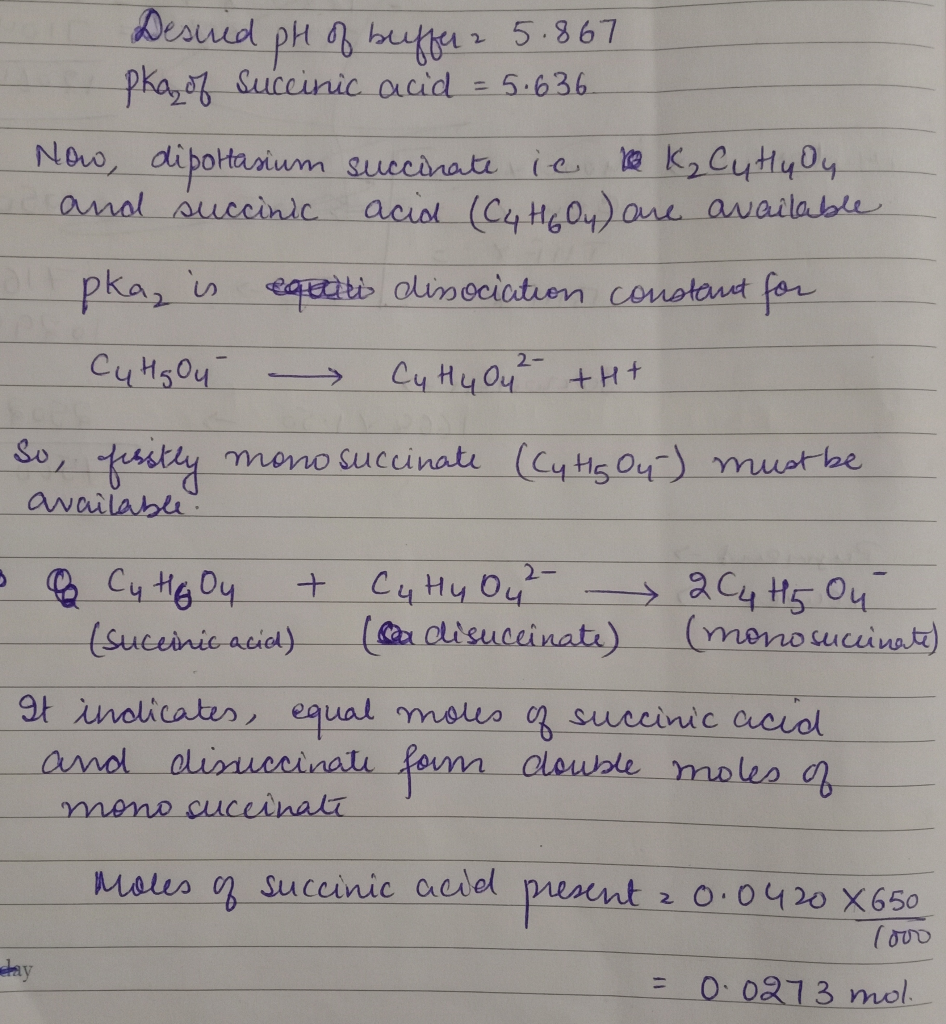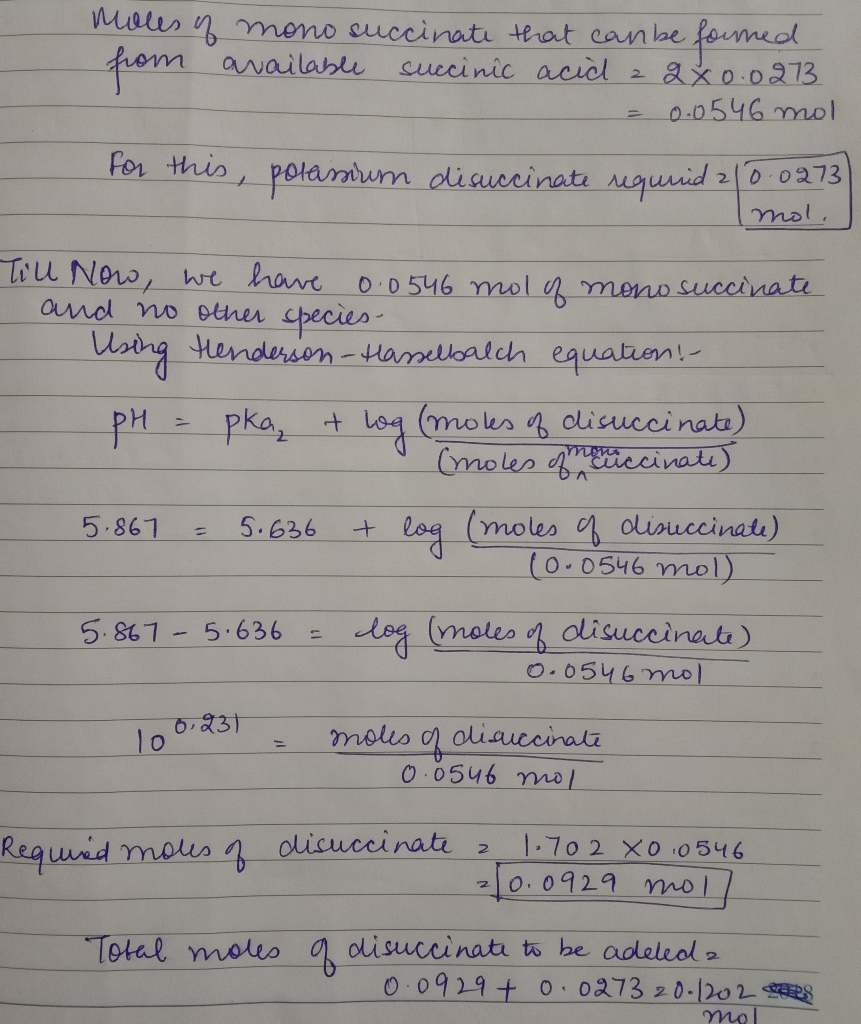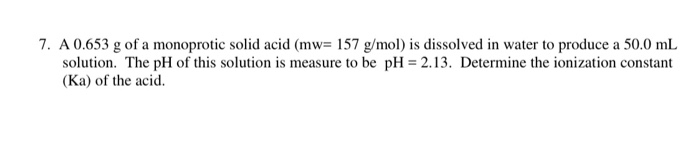Question

How many grams of dipotassium succinate trihydrate (K2C4H4O4·3H2O, MW = 248.32 g/mol) must be added to 650.0 mL of a 0.0420 M succinic acid solution to produce a pH of 5.867? Succinic acid has pKa values of 4.207 (pKa1) and 5.636 (pKa2).Molar mass of potassium disuccinate = 248.32 g/mol

Moles of potassium disuccinate required = 0.1202 mol

Mass of potassium disuccinate required

= 0.1202 mol*248.32g/mol

= 29.848 g

#### Earn Coins

Coins can be redeemed for fabulous gifts.

Similar Homework Help Questions
• ### How many grams of dipotassium phthalate (242.3 g/mol) must be added to 100 mL of 0.125...

How many grams of dipotassium phthalate (242.3 g/mol) must be added to 100 mL of 0.125 M potassium hydrogen phthalate to give a buffer of pH 5.80? The Ka's for phthalic acid are 1.12 x 10-3 and 3.90 x 10-6.

• ### Calculate the volume, in liters, of 1.548 M KOH that must be added to a 0.117...

Calculate the volume, in liters, of 1.548 M KOH that must be added to a 0.117 L solution containing 10.78 g of glutamic acid hydrochloride (H3Glu Cl–, MW = 183.59 g/mol) to achieve a pH of 10.36. Glutamic acid (Glu) is an amino acid with pKa values of pKa1 = 2.23, pKa2 = 4.42, pKa3 = 9.95.

• ### (c) (Challenging). 1.3608 g of sodium acetate trihydrate solid C2H3NaO2●3H2O (NaAc●3H2O, MM = 136.08 g/mol) was...

(c) (Challenging). 1.3608 g of sodium acetate trihydrate solid C2H3NaO2●3H2O (NaAc●3H2O, MM = 136.08 g/mol) was fully dissolved in 100.0 mL of pure water. What was the pH of this solution?

• ### How much volume of a 0.102 M NaOH solution must be added to 25.00 ml of...

How much volume of a 0.102 M NaOH solution must be added to 25.00 ml of a 0.075 M solution of benzene-1,2,3-tricarboxylic acid to obtain a pH equal to 4.80? pKa values: pKa1 = 2.86, pka2 = 4.30 and pka3 = 6.28.

• ### How many grams of KCN (Mm = 65.1 g/mol) must be added to 900. mL of...

How many grams of KCN (Mm = 65.1 g/mol) must be added to 900. mL of 1.240-M solution of HCN in order to prepare a pH = 8.86 buffer?

• ### 10.00 grams of KH2PO4 (FW = 136.086 g/mol) are dissolved in 500 mL of deionized H2O....

10.00 grams of KH2PO4 (FW = 136.086 g/mol) are dissolved in 500 mL of deionized H2O. How many grams Na2HPO4 (FW = 141.96 g/mol) need to be dissolved to create a solution with a pH of 6.90? For H3PO4, pKa1 = 2.15 , pKa2 = 7.20, and pKa3 = 12.37.

• ### 7. A 0.653 g of a monoprotic solid acid (mw= 157 g/mol) is dissolved in water...7. A 0.653 g of a monoprotic solid acid (mw= 157 g/mol) is dissolved in water to produce a 50.0 mL solution. The pH of this solution is measure to be pH = 2.13. Determine the ionization constant (Ka) of the acid. 8. A buffer is 0.50 M CH3COOH(aq) and 0.50 M Na(C6H-C00) (aq). For CH2COOH pKa = 4.20 a) Calculate the pH of the buffer solution. b) Calculate the pH after the addition of 40 mL of a 0.150...

• ### How many grams (to the nearest 0.01 g) of NH4Cl (Mm = 53.49 g/mol) must be...

How many grams (to the nearest 0.01 g) of NH4Cl (Mm = 53.49 g/mol) must be added to 550. mL of 0.976-M solution of NH3 in order to prepare a pH = 9.15 buffer? What volume (to the nearest 0.1 mL) of 4.00-M NaOH must be added to 0.600 L of 0.300-M HNO2 to prepare a pH = 3.50 buffer?

• ### How many grams (to the nearest 0.01 g) of NH4Cl (Mm = 53.49 g/mol) must be...

How many grams (to the nearest 0.01 g) of NH4Cl (Mm = 53.49 g/mol) must be added to 750. mL of 1.188-M solution of NH3 in order to prepare a pH = 9.90 buffer?

• ### How many grams (to the nearest 0.01 g) of NH4Cl (Mm = 53.49 g/mol) must be...

How many grams (to the nearest 0.01 g) of NH4Cl (Mm = 53.49 g/mol) must be added to 750. mL of 0.928-M solution of NH3 in order to prepare a pH = 8.95 buffer?